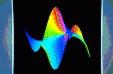This is a page of the former Algo team's web site. It won't be updated any longer.# Research Topics of the Algorithms Project

The primal objective of this project is the design, analysis, and optimization of high-performance algorithms in selected areas of computer science. Our methodology relies on a precise quantification of complexity phenomena associated to fundamental discrete mathematical structures, with main focus on combinatorics and computer algebra. Our activity in combinatorial algorithms is based on the development of a general theory known as analytic combinatorics. It finds spectacular applications, in random generation, quantitative data-mining, as well as the systematic treatment of complex combinatorial models. Our activity in computer algebra is targeted at the effective manipulation of special functions and complex functional systems. These objects are of central importance in analytic combinatorics, symbolic computation, as well as many other areas like mathematical physics, theoretical chemistry, and applied mathematics at large. Analysis (specifically, in the technical sense of functions of complex variables) plays a pivotal role, shared by both axes. The tight coupling of the two themes, combinatorial algorithms and symbolic algorithms, constitutes the distinguishing feature of the Algorithms project.

DISCRETE COMBINATORIAL STRUCTURES are ubiquitous in computer science and technology. For instance, the description of a discretized surface in three-dimensional space combines geometric information (point locations) with purely topological--combi\-natorial data; compressing efficiently a large text necessitates extracting intricate combinatorial patterns of replications. In both cases, efficient algorithms can be designed based on the elucidation of fine combinatorial properties of discrete objects, together with a quantification of which structures are typically likely to appear: ''metric'' properties of discrete structures can be exploited to distill high-performance algorithms. On an other register, communication protocols, such as the Ethernet, serve to regulate the interaction of a large number of agents, each described by extremely simple combinatorial rules; but getting a grasp on their collective, competitive, behaviour is far from trivial. There is a high payoff, though, and we have successfully showed how a suitable theory could be put to use in order to design protocols which exploit the capacity of a channel in a way close to its limits, while exhibiting stability properties much stronger than those of the Ethernet.

The areas mentioned above all benefit from an emerging global theory, called analytic combinatorics. The ambitious goal is to determine some of the major laws governing random discrete structures of large size, set up the fundamental equations of the field, and provide constructive theorems and methods aimed at obtaining effective solutions. Analytic combinatorics can thus be viewed as an operational calculus of discrete structures, one that offers an attractive and powerful alternative to more traditional probabilistic approaches. The analytic-combinatorial approach is then being developed in order to systematize the analysis of a very large variety of discrete algorithms and data structures of computer science as well to provide a sound basis for their design and optimization. Indeed, the systematic character of the quantitative analysis of discrete systems afforded by analytic combinatorics has led our team to investigate the possibility of treating complex combinatorial models by means of symbolic manipulation systems, also known as computer algebra and discussed next.

COMPUTER ALGEBRA is devoted to the study of effective mathematics and the complexity of corresponding algorithms. The emphasis is on exact mathematical objects (integers, rational numbers, polynomials, algebraic numbers,&dots;) rather than numerical approximations; the foundations lie in algebra. So far, most of the impact of this area has been in pure mathematics and in education. The applications of computer algebra systems such as Maple or Mathematica to applied, discrete or experimental mathematics have often been restricted by either the scarceness of exact solutions or the huge memory requirements on practical problems. Our long-term project aims at improving this state of affairs and bringing computer algebra to a stage where it can generate efficient and guaranteed numerical code for approximating the solutions of large classes of problems of real-life size stemming from analysis and combinatorial models. It has been realized (in a large part by members of the project), that many classical functions of mathematics and mathematical physics can be dealt with automatically in a computer algebra system provided one considers linear differential equations as a data-structure. The required tools there are non-commutative analogues of the algorithms used for polynomials. To give an idea of the impact of this approach, it can be estimated that approximately 60% of Abramowitz and Stegun's Handbook of Mathematical Functions (one of the most cited references in mathematics, physics, chemistry and engineering sciences) can be generated automatically by computer algebra programs using systems of linear differential equations as the basic data-structure. We also aim at applying this principle to the generation of good approximations for otherwise difficult to evaluate multiple integrals arising in theoretical physics or chemistry. In terms of efficiency, the regular increase of speed of modern computers is such that theoretical complexity analyses are more and more relevant in this area. Indeed, when computing times exceed a handful of seconds, the asymptotic regimes of the algorithms has often been reached. There is thus a renewal of activity in the design of quasi-optimal algorithms, relying on systematic algorithm design principles and a good control on the size of data-structures for intermediate mathematical objects. This area is both of a fundamental interest and crucial for achieving the desired efficiency in making algorithmic large fragments of computational complex analysis.

In summary, the Algorithms project has a strong theoretical basis, on which we graft the development of high performance algorithms in carefully selected areas, where analysis-driven design is likely to bring computational gains by factors often in the range 10--100. It combines a problem-specific opportunistic approach with long terms goals of a methodological nature.

For more on our topics of research, see our pages on